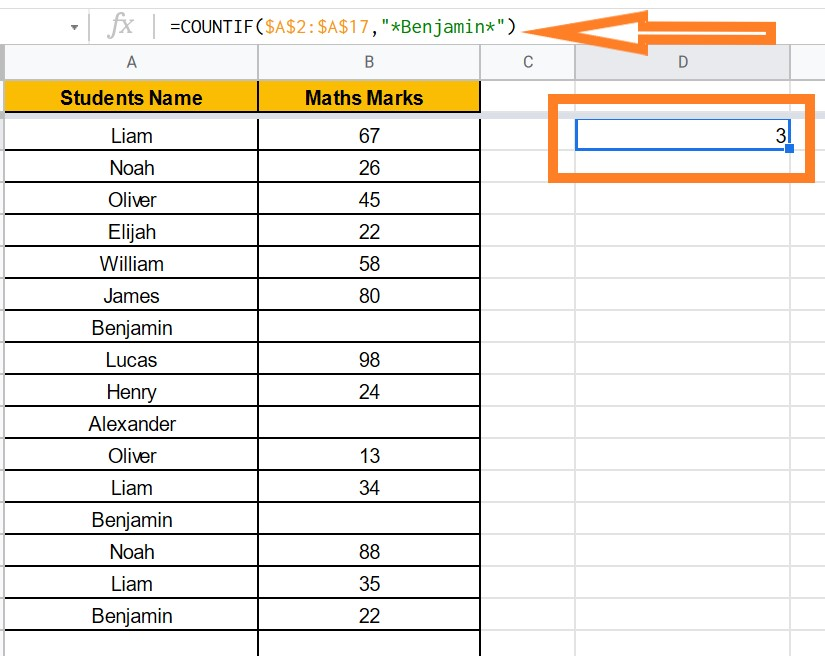## How to use COUNTIF Function in Google Sheets: If Cell Contains Text, Non Blank Cells

Google Sheets COUNTIF Functions is used when you want to count certain numbers or get a count of total numbers when certain conditions are met.

When the specified criterion is single, the COUNTIF function is used. For better understanding, The COUNTIF functions are divided into 2 parts, and they are as follows:

• IF Section: This section specifies the criteria that must be met for cells or data to be counted.
• COUNT Section: This function adds up the number of cells that meet the criteria.

## Google Sheet COUNTIF Function Formula

The COUNTIF function formula which needs to apply on Google sheets are given below:

=COUNTIF(range, criterion)

• range:  A range is a collection of cells that we need to find.
• Criterion: This should be the condition that needs to be met. Any criteria such as number, text, cell reference, expression can be used as a criterion when specifying the range.

When defining the criterion in the COUNTIF function, there are some guidelines to follow depending on whether the data range contains numeric or text values, and they are:

1. If the range contains numbers, then the criteria can be comparing operators such as >,<,=, <>, etc.
2. If the range contains the text, then it should be enclosed with double quotes. For example, if the text is apple, it should be enclosed with double quotes such as “apple”.
3. In the case of a text criterion, you can also use wildcard characters. For example, you can use apple* as a criterion to count cells that contain apple or apples.

## How to use COUNTIF Function on Google Sheets?

Let’s understand how to use Google sheets COUNTIF functions with an example.

### Google Sheets Count If Cell Contains Number

Let’s consider the following dataset.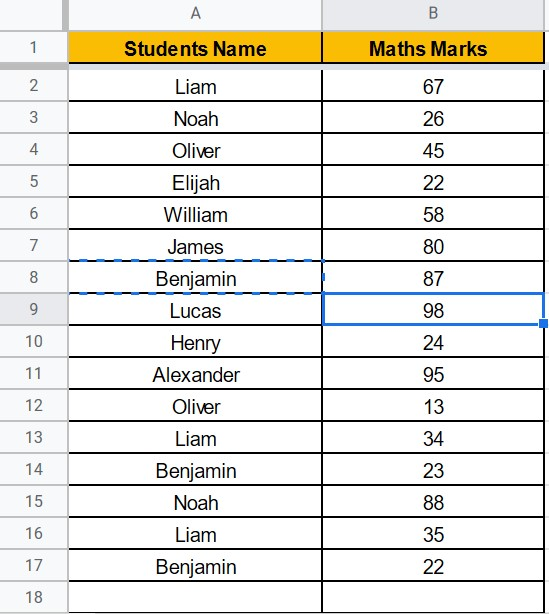Now let’s count the number of students who scored higher than 75 marks.

• Step 1: Select the Cell Range where you to get the count.
• Step 2: Now enter the formula “=COUNTIF(\$B\$2:\$B\$17,“>75”)”.
• Step 3: Press “Enter“.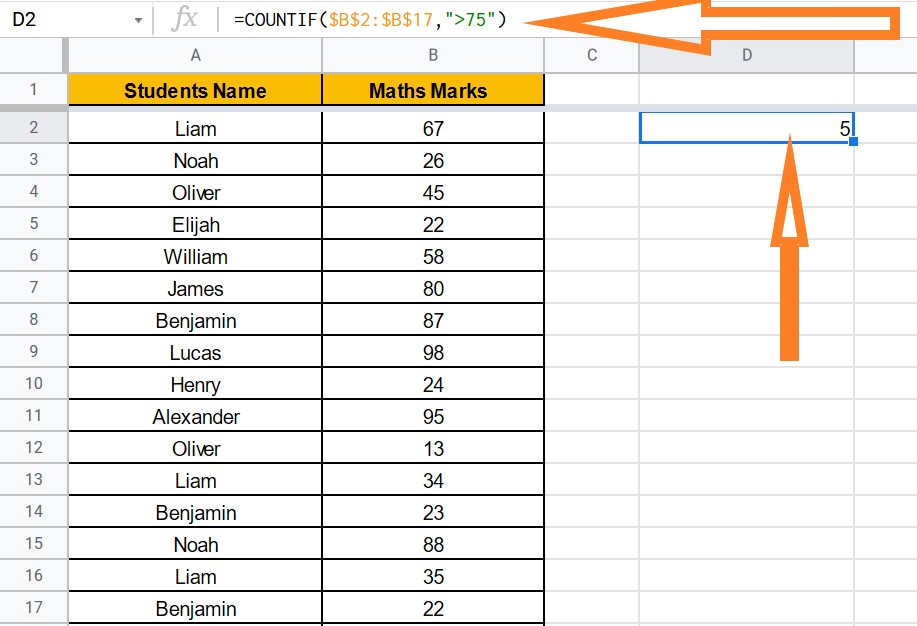The above result will be shown on the screen.

• To count the numbers whose marks is less than 75, use the formula as =COUNTIF(\$B\$2:\$B\$17,“<75”)
• To get the count whose marks are equal to 35, use the formula: =COUNTIF(\$B\$2:\$B\$17,“=35”)

### Google Sheets Count If Cell Contains Text

Let’s consider the following data set.I want to count the cell, which consists of the name “Benjamin“.

• Step 1: Select the cell where you would like to get the count.
• Step 2: Now enter the formula as “=COUNTIF(\$A\$2:\$A\$17,Benjamin)”
• Step 3: Click on “Enter“.

You will get the count with the names Benjamin.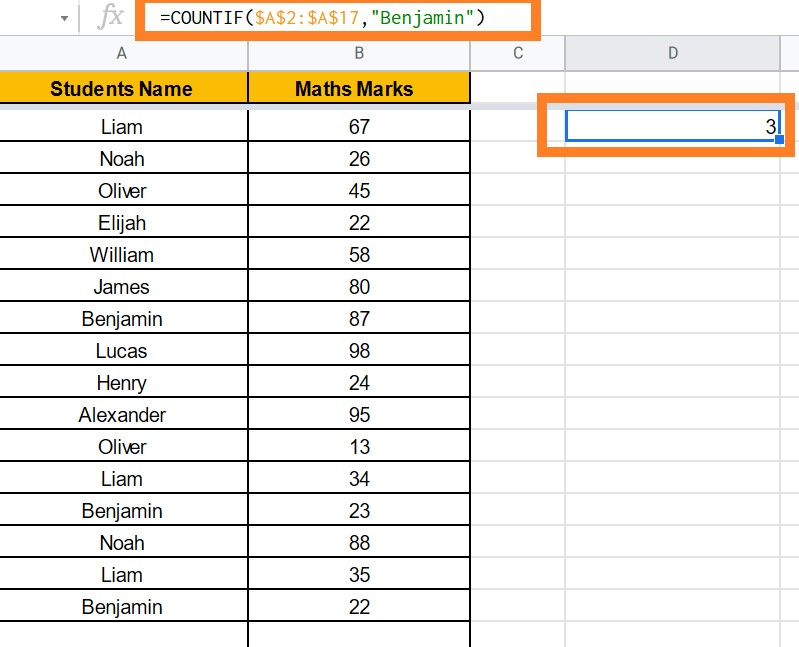### Google Sheets Count If Cell Does Not Contain Particular Text

Consider the above dataset as an example. Now, let’s count the number of cells that don’t consist of Benjamin’s name by following the steps listed below.

• Step 1: Select the cell where you want to get the count.
• Step 2: Enter the formula “=COUNTIF(\$A\$2:\$A\$17,“<>Benjamin”)“.
• Step 3: The results will be displayed on the screen as shown below.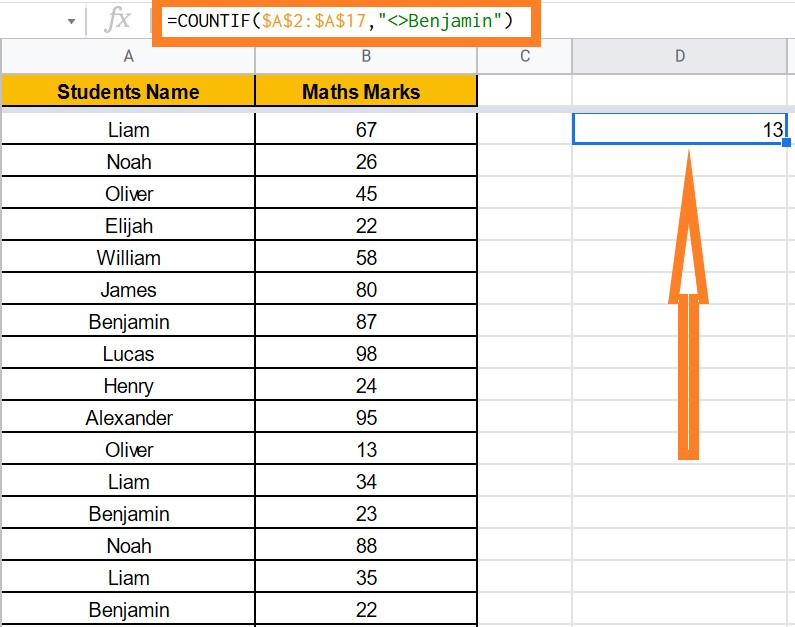### Google Sheets Count If for Blank or Empty Cells

Check our article How to Count Cells If Not Blank in Google Sheets which says everything about the following steps in detail.

• Step 1: Select the cell to get the count for empty cells
• Step 2: Enter the formula “=COUNTIF(\$B\$2:\$B\$17,“ ”)“.
• Step 3: The results will be displayed on the screen as shown below.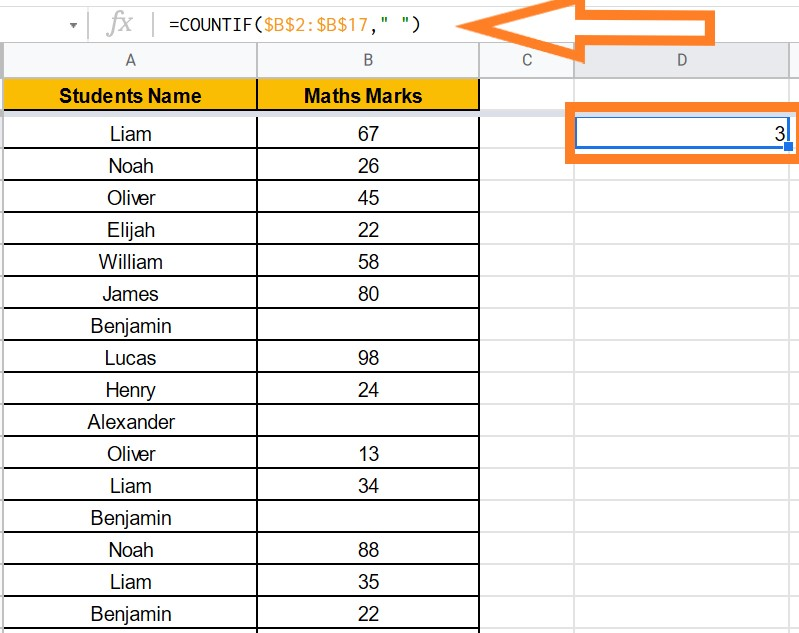### Google Sheets Count If for Non Empty Cells

• Step 1: Select the cell to get the count for non-empty cells
• Step 2: Enter the formula “=COUNTIF(\$B\$2:\$B\$17,“<>”)“.
• Step 3: The results will be displayed on the screen as shown below.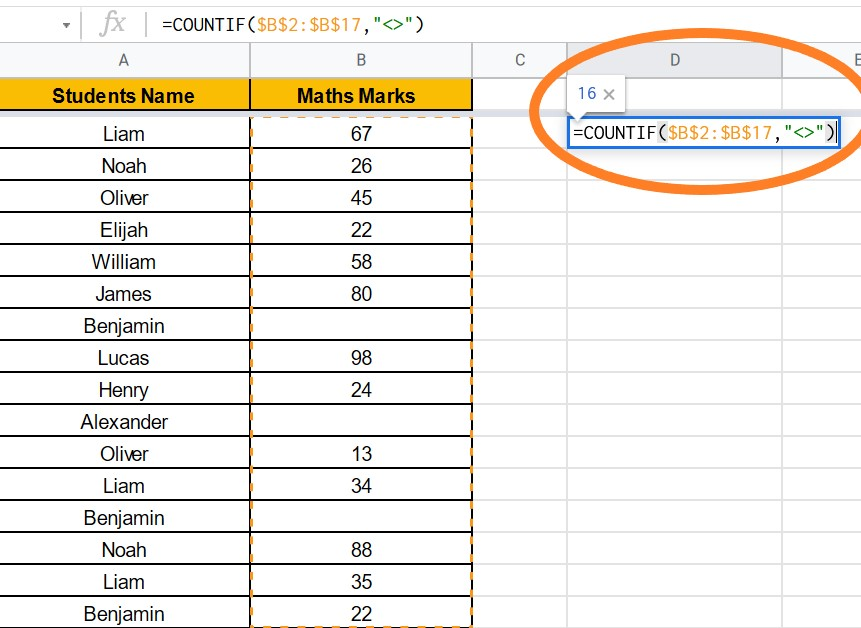### Google Sheets Count If where Keyword Appears

• Step 1: Select the cell to get the count for non-empty cells
• Step 2: Enter the formula “=COUNTIF(\$B\$2:\$B\$17,“*Benjamin*”)“.
• Step 3: The results will be displayed on the screen as shown below.